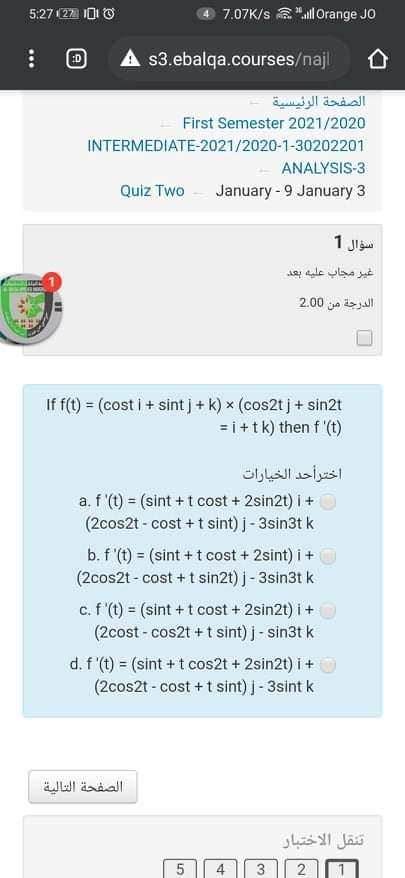### Create an Account

Home / Questions / 8 ال 122 5:27 7.07K/s "All Orange JO : (0) A s3.ebalqa.courses/najl الصفحة الرئيسية First ...

# 8 ال 122 5:27 7.07K/s "All Orange JO : (0) A s3.ebalqa.courses/najl الصفحة الرئيسية First Semester 2021/2020 INTERMEDIATE-2021/2020-1-30202201 ANALYSIS-3 Quiz Two January - 9 January 3 سؤال 1 غير مجاب

8 ال 122 5:27 7.07K/s "All Orange JO : (0) A s3.ebalqa.courses/najl الصفحة الرئيسية First Semester 2021/2020 INTERMEDIATE-2021/2020-1-30202201 ANALYSIS-3 Quiz Two January - 9 January 3 سؤال 1 غير مجاب عليه بعد الدرجة من 200 If f(t) = (cost i + sint + k) * (cos2t j + sin2t =i+tk) then f'(t) اختر أحد الخيارات a.f'(t) = (sint + t cost + 2sin2t) i + (2cos2t - cost + t sint) j - 3sin3tk b.f'(t) = (sint + t cost + 2 sint) i + (2cos2t - Cost + t sin2t) j - 3sin3tk c. f (t) = (sint + t cost + 2sin2t) i+ (2cost - cos2t + t sint) j - sin3tk d.f(t) = (sint + t cos2t + 2sin2t) i+ (2cos2t - cost + t sint) | - 3sint k الصفحة التالية تنقل الاختبار 5 5 4 3 21

May 11 2021 View more View LessSubscribe To Get Solution